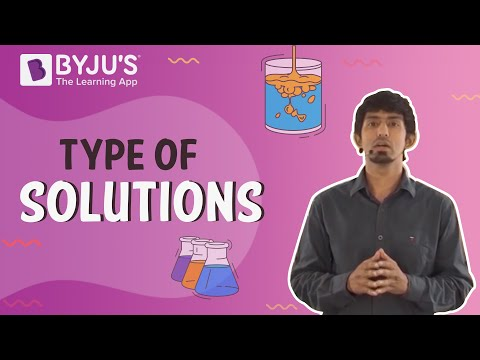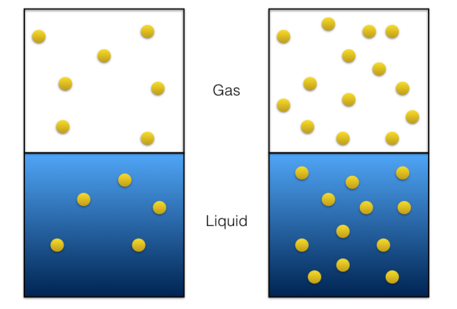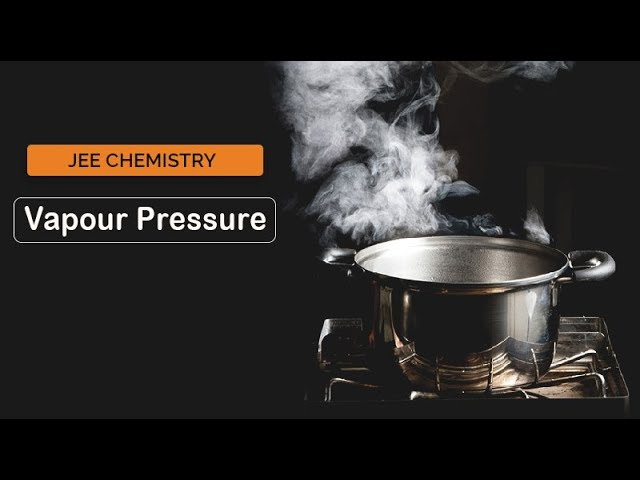# Solutions Class 12 Notes Chapter 2

A solution comprises a solute and a solvent. It is defined as a homogeneous mixture of two or more than two substances. They can be classified into three types:

• Solid solutions
• Gaseous solutions
• Liquid solutions

Molarity, mole fraction, percentages, and molality are the terms used to express the concentration of a solution.

Students can refer to the short notes and MCQ questions along with separate solution pdf of this chapter for quick revision from the links below:

#### For More Information On Types of Solutions, Watch The Below Video:## Henry’s law

In liquids, the dissociation of gas is controlled by Henry’s law. The states that, the solubility of a gas in a liquid, at a given temperature, is directly proportional to the partial pressure of the gas. Due to the presence of a non-volatile solute, the vapour pressure of the solvent is lowered.## Raoult’s Law

The lowering of the vapour pressure of the solvent is ruled by Raoult’s law. The law states that the relative lowering of the vapour pressure of the solvent over a solution and the mole fraction of a non-volatile solute present in the solution is equal. It is expressed as:

Ptotal= p10x1+p20x2

## Ideal Solutions

When a solution obeys Raoult’s law over the entire range of concentrations it is called an ideal solution. Types of deviations from Raoult’s law:

• Positive deviations
• Negative deviationsStudents can also read more from the

### Few Important Questions

1. What is mole fraction?
2. Explain the role of molecular interaction in a solution of water and alcohol?
3. Give the statement of Henry’s law. List some applications.
4. What is a solution? Explain the different types of solution.
5. What is molarity?

Browse the notes of Solutions Class 12 Chemistry for CBSE syllabus from BYJU’s to explore more about this chapter. Keep visiting us for latest updates on CBSE class 12 chemistry notes.

## Frequently asked Questions on CBSE Class 12 Chemistry Notes Chapter 2: Solutions

### What are the different types of solutions?

The 3 main types of solutions are: 1. Solid 2. Liquid 3. Gas

### What are ‘Ideal solutions’?

When a solution obeys Raoult’s law over the entire range of concentrations it is called an ideal solution.

### What is ‘Molarity’?

Molarity or molar concentration, is the concentration of a solution measured as the number of moles of solute per liter of solution.